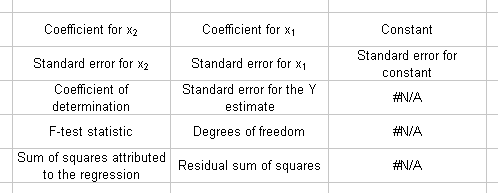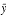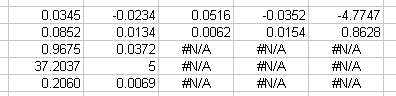# LINEST

Description Computes multiple linear regression for a group of observations relative to a number of independent variables. Also optionally computes statistics related to the regression.

Note This is an array function. For information, see Array Functions.

Syntax LINEST(known_y's [, known_x's] [, constant] [, statistics]
Argument Description
known_y's A list of observed values for the dependent variable. This must be a range reference or an array constant containing all numeric values and no empty cells. For a regression with a single independent variable, enter any type of range. For a regression with multiple independent variables, enter a single row or column of values. If you are using array constants, see Array Constants in Arguments for information on how to enter the array.
[known_x's] Optional. A list of observed values for the independent variable(s). This must be a range reference or an array constant containing all numeric values and no empty cells. For a regression with a single independent variable, when known_y's is a single row or column, enter a range that exactly matches the size and shape of the known_y's range. When known_y's is more than one row and column, known_x's must be a range containing the same number of values as the known_y's range, although the two ranges may be different shapes. For a regression with multiple independent variables, when known_y's is a single row, enter a contiguous row of values for each independent variable, The number of columns must match the number of columns in known_y's. Similarly, when known_y's is a single column, enter a contiguous column of values for each independent variable, ensuring that the number of rows matches known_y's. If you are using array constants, see Array Constants in Arguments for information on how to enter the array. To fit the regression to a formula that uses polynomials, see "Polynomials," below. When you omit this argument, the function assumes a single independent variable of the sequence {1, 2, 3, ...}.
[new_x's] Optional. A list of values for the independent variable(s) that will compute the results of this function. This must be a range reference or an array constant containing all numeric values and no empty cells. You may enter any number of values in new_x's. The shape of the range only matters if this is a regression with multiple independent variables, in which case it must have the same number of rows or colunms of independent variables as known_x's. If you omit this argument, the function uses the specified or default values in known_x's.
[constant] Optional. A logical value that determines whether or not a constant is to be included. The constant allows the regression line to intercept the Y axis at a point other than (0,0). Use True (the default value if this argument is omitted) to include the constant. Use False to force the line to intercept the Y axis at 0.

Results range Before entering this function, select a results range of the following size:

• It must have the same number of columns as your regression has independent variables, plus one. (If your regression has 3 independent variables, your results range must have 4 columns.)
• It must have 1 row if statistics is False, 5 rows if statistics is true.

Results map This map shows how LINEST fills the results range with data and statistics.In the last three statistics rows, for results ranges wider than three columns, #N/A will appear in the third and subsequent columns. This is correct.

Coefficients The coefficients computed by LINEST are displayed in the first row of the results range. For a multiple regression, the coefficients are displayed in the reverse order of the independent variables. (If your regression has 3 independent variables, the coefficient associated with the 1st variable is displayed in the 3rd column, the coefficient associated with the 2nd variable in the 2nd column, and the coefficient associated with the 3rd variable in the 1st column.)

The last cell of the first row of the results range displays the constant value, which will be 0 if constant is False.

If there is only one independent variable and constant is True, then the two coefficients will equal the results of the SLOPE and INTERCEPT functions when calculated on the same data.

Coefficients of 0 indicate either that there are not enough data points for the number of independent variables, or that some of the independent variables are too closely related.

Equation The regression computed by LINEST attempts to fit the following formula:

y = C1x1+C2x2+ ... + CnXn+b

where C is the coefficient, n is the number of independent variables, and b is the constant.

Statistics If statistics is True, the results range displays the following statistics in the following locations.
 Standard Error Values row 2 The error values correspond to the coefficients displayed directly above them. This is computed by dividing up the standard error for the Y estimate (see below) into components for each independent variable. It is an estimate of how much the errors in individual independent variable measurements contribute to the overall error. Coefficient of determination row 3, col 1 This statistic is similar in concept to the correlation coefficient computed by the RSQ function. It is a number between 0 and 1 that measures goodness of fit: low numbers indicate a poor fit, high numbers a good fit. Standard error for the Y estimate row 3, col 2 This is computed by taking the square root of the residual sum of squares (see below) divided by the degrees of freedom (see below). F-test statistic row 4, col 1 This statistic can be used with FINV to do null hypothesis testing on the overall goodness of fit of the regression. Degrees of Freedom row 4, col 2 This is determined by subtracting the number of independent variables from the number of values in known_y's, less 1 if constant is True. This statistic is used when running FINV and TDIST on the data to judge goodness of fit of the formula. Sum of squares attributed to the regression row 5, col 1 This is determined by subtracting the residual sum of squares (below) from the total sum of squares of the differences between the values in known_y's and. This statistic helps determine how well the formula fits the data. Residual sum of squares row 5, col 2 This is determined by summing the squares of the differences between the values in known_y's and the Y values computed by the formula you are trying to fit. It is a measure of the goodness of fit.

Using Statistics A user who wishes to determine if the formula returned by LINEST is a good fit for the data in the regression should perform the following two tests.

• Overall goodness of fit. To determine if the overall regression is a good fit, you can do null hypothesis testing using the F-test statistic returned by LINEST. Compare the F-test statistic to the F-rejection value calculated by running FINV with the probability of your choice (usually .01 or .05), and a denominator value of the degrees of freedom statistic returned by LINEST.
If the F-test statistic is larger than the F-rejection value, then the null hypothesis is rejected and this is a good fit.
• Goodness of fit of each independent variable. After determining that the overall regression is a good fit, test each independent variable to determine whether it adds to the soundness of the regression as a whole. Do this by finding the T-distribution of the T-observed statistic. The T-observed statistic is computed by dividing the coefficient of the variable you want to test by its standard error.
Find the T-distribution by running TDIST using the absolute value of T-observed, the degrees of freedom statistic returned by LINEST, and a one-tailed distribution.
If the probability returned by TDIST is larger than the rejection criteria (usually 0.01 or 0.05), then the variable you tested may not be contributing to the regression.

Polynomials To create a polynomial regression, the data for the independent variable must be set up carefully. Create a worksheet in which column A represents x and contains the appropriate data or formulas. For column B, which will contain x2, fill the cells with a formula such as `=A1^COLUMN()`, which will raise the data in column A to the 2nd power. Fill column C with the same formula to raise the data in column A to the 3rd power.

Example The following example of multiple linear regression attempts to correlate physiological data on preadolescent boys with their maximal oxygen uptake. The data are given in the table below.
 Maximal O2 Uptake y Age (years) x1 Height (cm) x2 Weight (kilos) x3 Chest Depth (cm) x4 1.54 8.4 132.0 29.1 14.4 1.74 8.7 135.5 29.7 14.5 1.32 8.9 127.7 28.4 14.0 1.50 9.9 131.1 28.8 14.2 1.46 9.0 130.0 25.9 13.6 1.35 7.7 127.6 27.6 13.9 1.53 7.3 129.9 29.0 14.0 1.71 9.9 138.1 33.6 14.6 1.27 9.3 126.6 27.7 13.9 1.50 8.1 131.8 30.8 14.5

The data were entered in a worksheet, the LINEST function was run, and the following results range was returned.